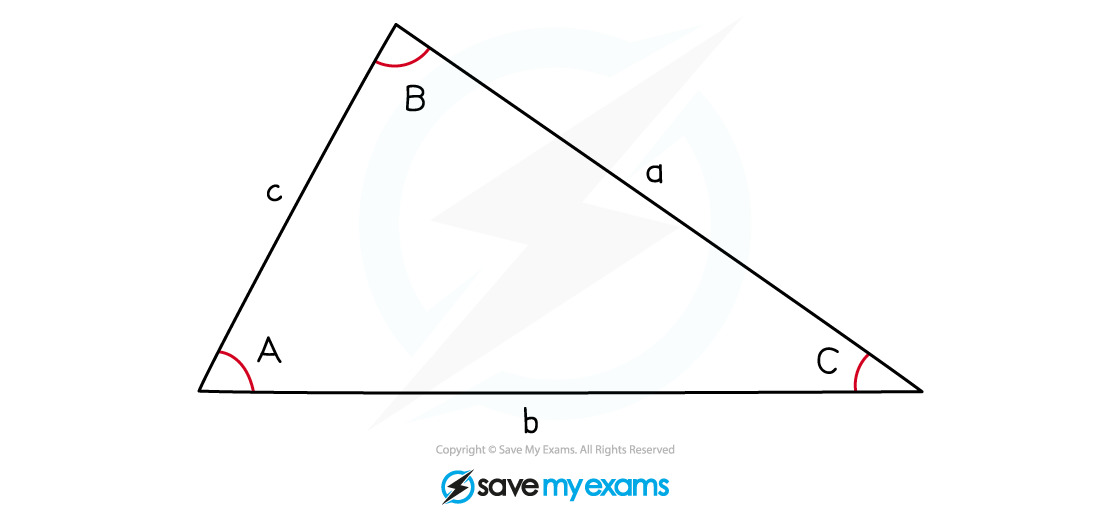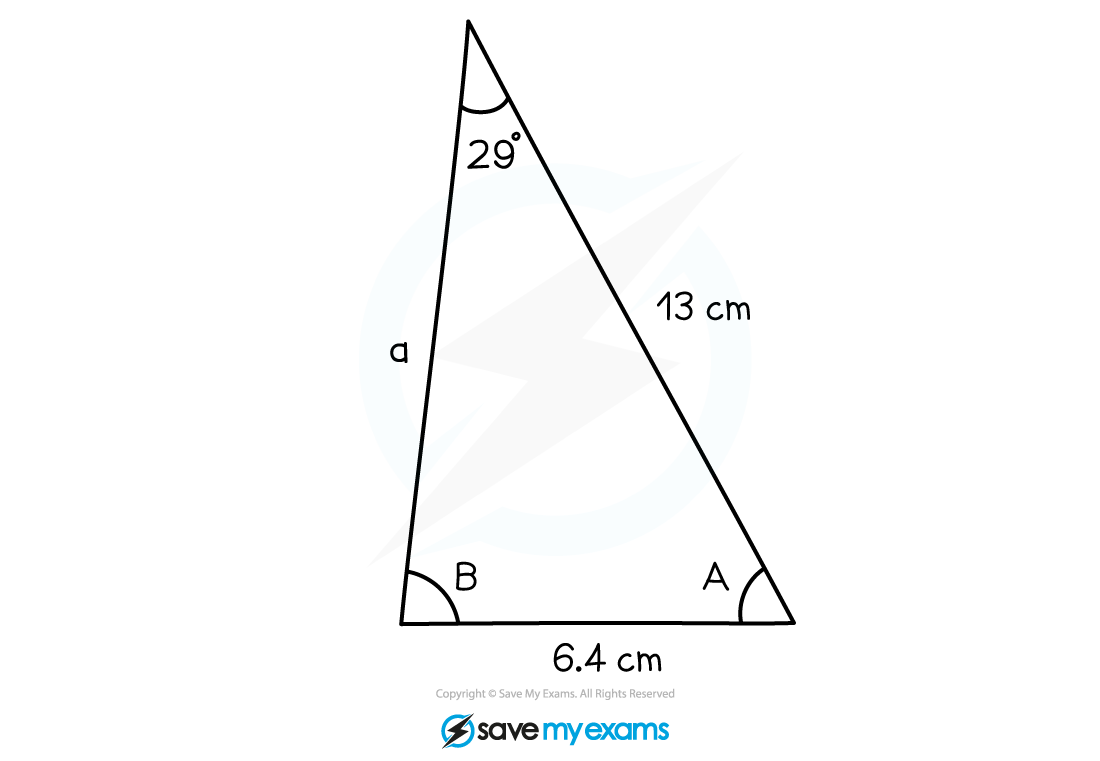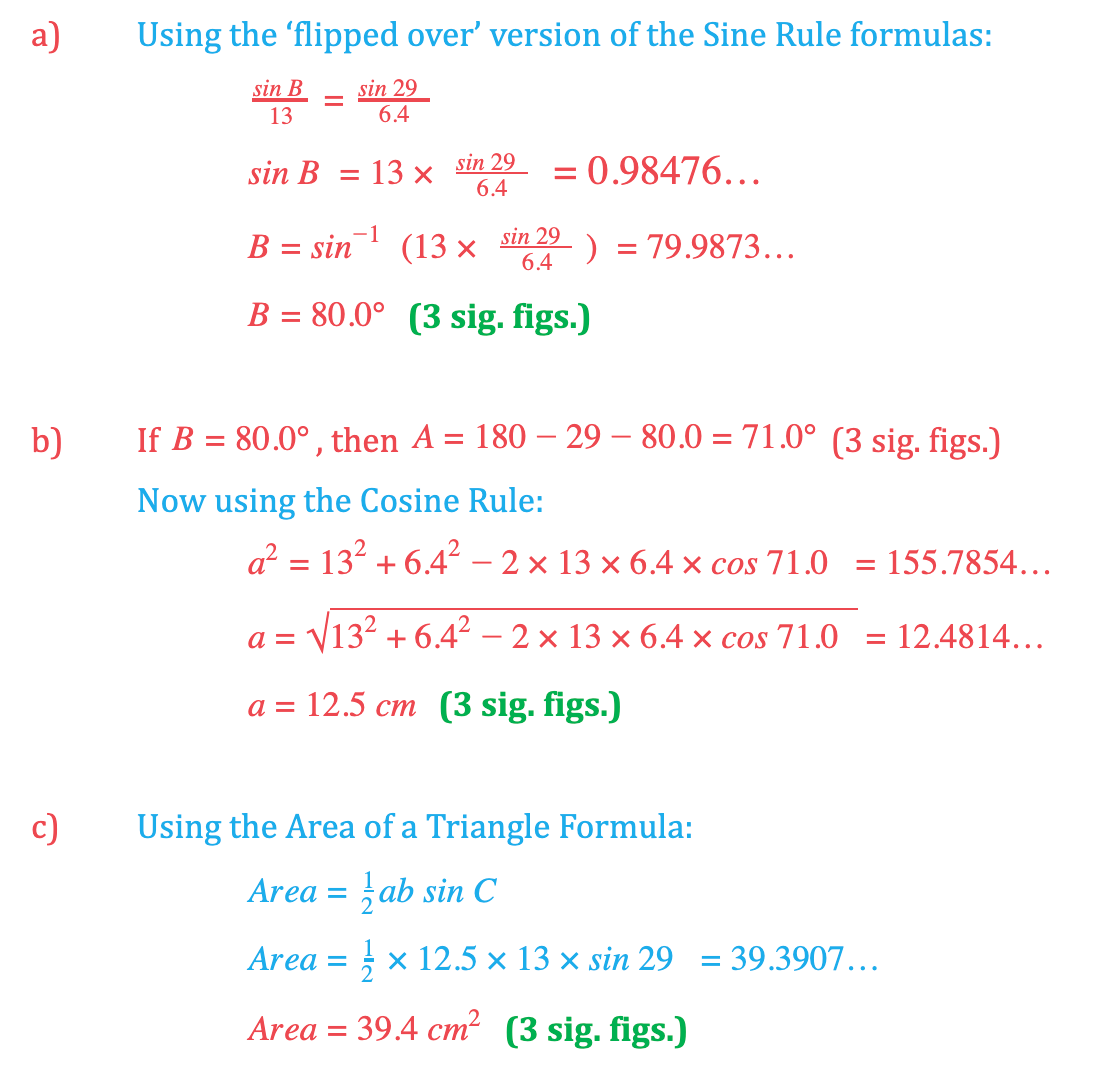# Edexcel IGCSE Maths 复习笔记 4.10.1 Sine & Cosine Rules, Area of a Triangle - Basics

Edexcel IGCSE Maths 复习笔记 4.10.1 Sine & Cosine Rules, Area of a Triangle - Basics

#### What are the sine & cosine rules, & the area of a triangle formula?

• Remember, Pythagoras and SOHCAHTOA only work in right-angled triangles
• The Sine RuleCosine Rule, and Area of a Triangle Formula allow us to answer triangle questions for ANY triangle

#### The sine rule, cosine rule, & area of a triangle formula

• In discussing these formulas, we usually label our triangle like this:• Note: lowercase letters for side lengths, capital letters for angles — and make sure an angle and the side opposite it have the same letter
• The Sine Rule tells us that:sin 90° =1 – so if one of the angles is 90°, this becomes ‘SOH’ from SOHCAHTOA

• The Cosine Rule tells us that:
`a2 = b2 + c2 – 2bc cos A`

cos 90° = 0   so if A = 90°, this becomes Pythagoras’ Theorem

• The Area of a Triangle Formula tells us that:sin 90° =1   so if C=90°, this becomes Area = ½ × base × height
`Area = ½ ab sin C`

• The Sine Rule can also be written ‘flipped over’:• This is more useful when we are using the rule to find angles
• These two versions of the Cosine Rule are also valid for the triangle above:

b2 = a2 + c2 - 2ac cos B

c2 = a2 + b2 - 2ab cos C

Note that it’s always the angle between the two sides in the final term

• These two versions of the Area of a Triangle Formula are also valid for the triangle above:Note that it’s always the angle between the two sides on the right-hand side –you can always just relabel the sides and angles of your triangle, though, instead of worrying about alternate versions of the last two formulas

#### Worked Example#### Worked Example